Preliminary results as of Summer 2012
(ICHEP 12 conference)

 The global CKM fit: Inputs and Numerical results The global CKM fit in the large (ρ-bar,η-bar) plane Constraint from BR(B+→τ+ ν) New physics in neutral-meson mixing Branching ratio of Bs→μ+ μ- The global CKM fit in the small (ρ-bar,η-bar) plane (zoom) The global CKM fit in the large (ρs-bar,ηs-bar) plane The global CKM fit in the (|Vud|,|Vus|) plane The global CKM fit in the (|Vcd|,|Vcs|) plane Constraints on the angle α/ϕ2 from charmless B decays Constraints on the angle γ/ϕ3 from B decays to charm Constraints on |sin(2β+γ)|

Numerical results:

 The results of the global CKM analysis include: Wolfenstein parameters, UT angles and sides, UTsangle and apex, CKM elements, theory parameters, rare branching fractions (B->lν, B->ll).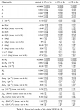Numerical Results

Constraints in the (ρ-bar,η-bar) plane. The red hashed region of the global combination corresponds to 68% CL.
 α, β, γconvention ϕ1, ϕ2, ϕ3convention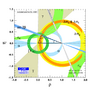eps png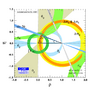eps png
Constraints in the (ρ-bar,η-bar) plane. The |Vub| constraint has been split in the two contributions: |Vub| from inclusive and exclusive semileptonic decays (plain dark green) and |Vub| from B+→τ+ ν (hashed green). The red hashed region of the global combination corresponds to 68% CL.
 α, β, γconvention ϕ1, ϕ2, ϕ3convention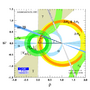eps png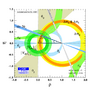eps png
One notices that the ring coming from the combined constraint from Δ md and Δ ms is cut for values of ρ close to 2. This can be understood by the fact that the two constraints provide ranges respectively on

|Vtd|2=A2 λ6 [(1-ρ)2+η2+O(λ4)]

|Vts|2=A2 λ4 [1-λ2(1-2 ρ)+O(λ4)]

The 68% and 95% CL constraints on ρ from Δ ms are indicated in the two shades of red, together with the (circular) constraint from Δ md and the mended ring once the two constraints are combined.
 α, β, γconvention ϕ1, ϕ2, ϕ3convention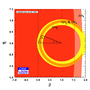eps png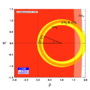eps png

A new value of BR(B→τν) from Belle has led to a decrease of the world average. The discrepancy in the CKM global fit between the world averages for sin2β and BR(B→τν) has thus been eased significantly.
There is a specific correlation between the two quantities in the global fit, which is best viewed in the (sin2β,BR(B→τν)) plane, regarding the prediction from the global fit without using these measurements. The cross corresponds to the experimental value with 1 sigma errors.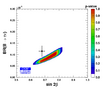eps png

The shape of the correlation can be understood by considering the ratio BR(B→τν)/Δmd, where the decay constant fBd cancels, leaving limited theoretical uncertainties (the ratio depends only on the bag parameter BBd). Thus from the observables BR(B→τν) and Δmd one gets an interesting constraint in the (ρbar,ηbar) plane.
 α, β, γconvention ϕ1, ϕ2, ϕ3convention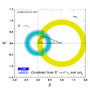eps pngeps png
To have a closer look, one can write the full formula for the ratio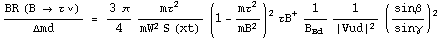where one explicitly sees that the correlation between BR(B→τν) and the angle β is controlled by the values of BBd, and the angles α and γ. This can be checked explicitly by comparing the above analytical formula with the colored region in the (sin2β,BR(B→τν)) plane.

To quantify the discrepancy one can compare the indirect fit prediction for BR(B→τν) with the measurement. The deviation here is 1.6 sigmas.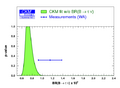eps png

A simpler test is the comparison of the prediction of BBd from the above analytical formula BBd = 0.80+0.23-0.16 (having only BR(B→τν), Δmd, α, β, γ and |Vud| as inputs, that is an almost completely theory-free determination of BBd), with the current lattice determination BBd = 1.29 +0.08-0.08. For this test the deviation is 1.4 sigmas.eps pngIndividual constraints correspond to 68% CL (see: arXiv:1008.1593 [hep-ph] and arXiv:1203.0238. [hep-ph] for a detailed explanation of the hypotheses). These plots have been obtained with the following updates: (φs,ΔΓs) likelihood provided by HFAG averaging D0, CDF and LHCb results for Bs→J/Ψ φ, the new HFAG average for aSLd=0.0038±0.0036 the average of the LHCb [LHCb-CONF-2012-022] and D0 [0904.3907] results for aSLs=-0.0022±0.0052.
In scenario I we have introduced NP in M12q = M12SM,qΔq independently for Bd, Bs and K, corresponding to NP with arbitrary flavour structure.

Constraints on New Physics in the (ReΔd,ImΔd) plane. A 1.6 σ deviation is obtained for the 2-dimensional SM hypothesis Δd = 1 (Re(Δd)=1, Im(Δd)=0).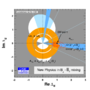eps png

Constraints on New Physics in the (ReΔs,ImΔs) plane. A 0.2 σ deviation is obtained for the 2-dimensional SM hypothesis Δs = 1 (Re(Δs)=1, Im(Δs)=0).

The pulls for the relevant observables are 0.1 σ for B->τν, 2.8 σ for φsJ/Ψ Φ and 3.3 σ for ASL, illustrating the difficulty to accomodate the last two results in this scenario.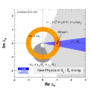eps png

Indirect constraint on the asymmetry ASL=(-7.5+5.0-6.3)x10-4 or (44.7+9.7-7.1)x10-4, compared with the direct measurements of CDF and D0. A second solution is now allowed due to the update of the input for aSLd.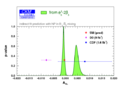eps png
Indirect constraint on the CP phase φsJ/Ψ Φ=-61.8+10.5-7.1 degrees, compared with the direct measurements of CDF, D0 and LHCb.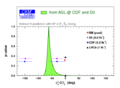eps png
 Prediction for Br(Bs→μ+ μ-)=(3.63+0.21-0.34)x10-9. The blue (respectively red) curve represents the prediction removing the input from Δ ms (respectively fBs). The red curve is actually identical to the green one, indicating that the prediction is dominated by the indirect determination of fBs through the global fit (more specifically Δms), and not by its direct input.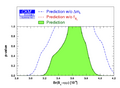eps png

Zoomed constraints in the (ρ-bar,η-bar) plane.The red hashed region of the global combination corresponds to 68% CL.
 α, β, γconvention ϕ1, ϕ2, ϕ3convention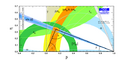eps png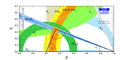eps png
Zoomed constraints in the (ρ-bar,η-bar) plane. The |Vub| constraint has been split in the two contributions: |Vub| from inclusive and exclusive semileptonic decays (plain dark green) and |Vub| from B+→τ+ ν (hashed green). The red hashed region of the global combination corresponds to 68% CL.
 α, β, γconvention ϕ1, ϕ2, ϕ3convention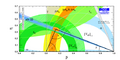eps png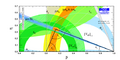eps png
Zoomed constraints in the (ρ-bar,η-bar) plane not including the angle measurements in the global fit.
 α, β, γconvention ϕ1, ϕ2, ϕ3convention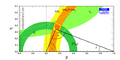eps png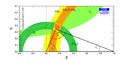eps png
Constraints in the (ρ-bar,η-bar) plane including only the angle measurements.
 α, β, γconvention ϕ1, ϕ2, ϕ3convention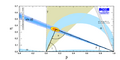eps png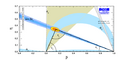eps png
Constraints from CP conserving quantities (|Vub / Vcb|, Δmd, (Δmd and Δms) and B+ →τ+ ν) in the (ρ-bar,η-bar) plane.
 α, β, γconvention ϕ1, ϕ2, ϕ3convention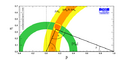eps png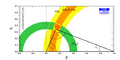eps png
Constraints from CP violating quantities (sin(2β), α, γ and εk) in the (ρ-bar,η-bar) plane.
 α, β, γconvention ϕ1, ϕ2, ϕ3convention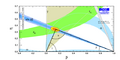eps png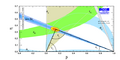eps png
Constraints from "Tree" quantities in the (ρ-bar,η-bar) plane (γ(DK) and α from the isospin analysis with the help of sin2β (charmonium), which gives another tree only γ measurement (the only assumption is that the ΔI=3/2 b-->d EW penguin amplitude is negligible)).
 α, β, γconvention ϕ1, ϕ2, ϕ3convention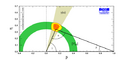eps png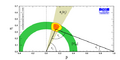eps png
Constraints from "Loop" quantities in the (ρ-bar,η-bar) plane.
 α, β, γconvention ϕ1, ϕ2, ϕ3convention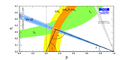eps png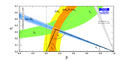eps png
Constraints in the (ρ-bar,η-bar) plane, not including the braching ratio of B+ → τ+ν in the global fit.
 α, β, γconvention ϕ1, ϕ2, ϕ3convention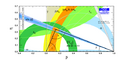eps png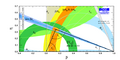eps png
Constraints in the (ρ-bar,η-bar) plane not including the measurement of sin2β in the global fit.
 α, β, γconvention ϕ1, ϕ2, ϕ3convention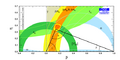eps png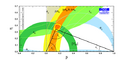eps png

The global CKM fit in the (|Vud|,|Vus|) plane:

 Constraints in the (|Vud|,|Vus|) plane. The indirect constraints (coming from b transitions) are related to |Vud| and |Vus| through unitarity.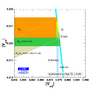eps png

 Constraints in the (|Vcd|,|Vcs|) plane. The indirect constraints (coming from b and s transitions) are related to |Vcd| and |Vcs| through unitarity. The direct constraints combine leptonic and semileptonic D and Ds decays as well as information from neutrino-nucleon scattering and W → cs decays. The following plots correspond to different subsets of these constraints.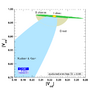eps png Constraints in the (|Vcd|,|Vcs|) plane where direct constraints involve only leptonic D and Ds decays with our inputs for lattice averages fDs/fD=1.185 ± 0.005 ± 0.010 and fDs=249.2 ± 1.2 ± 4.5 MeV.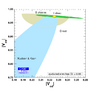eps png Constraints in the (|Vcd|,|Vcs|) plane where direct constraints involve only semileptonic D and Ds decays with our inputs for lattice averages FD → π(0)=0.666 ± 0.017 ± 0.048 and FD → K (0)=0.747 ± 0.010 ± 0.034.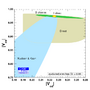eps png Constraints in the (|Vcd|,|Vcs|) plane where direct constraints involve only information from neutrino-nucleon scattering and W→ cs decays (no lattice input).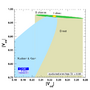eps png

Constraints on the angle α/ϕ2 from charmless B decays:

 No update See Winter 12 results (here).

 No update See Winter 12 results (here).

 No update See Winter 12 results (here).